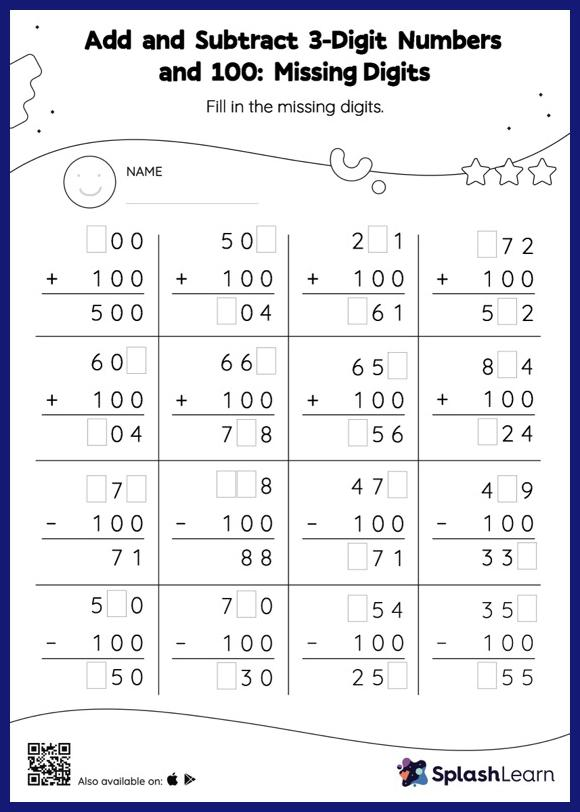# Add and Subtract 3-Digit Numbers and 100: Missing Digits Worksheet

Home > Add and Subtract 3-Digit Numbers and 100: Missing DigitsDoes your child know how to add and subtract 3-digit numbers and 100? Invite them to practice this concept here. In add and subtract 3-digit numbers and 100 worksheet, students must find the missing number using the count-on or count back method or the relationship between addition and subtraction. This worksheet is about practicing the column method in which numbers are written one on top of another. In this method, students use their place value understanding to solve the problems.